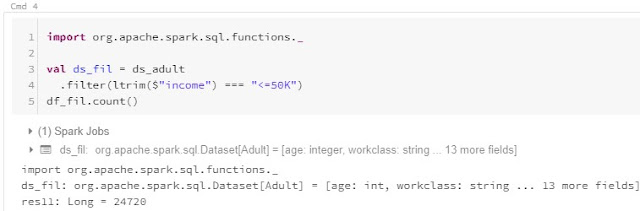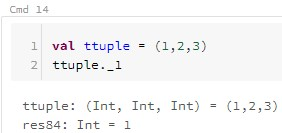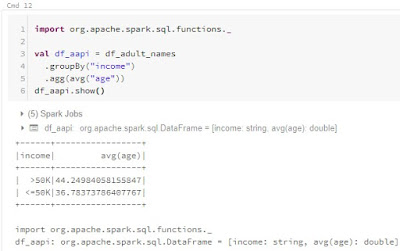## Monday, October 7, 2019

### Azure Databricks: RDDs, Data Frames and Datasets, Part 2

Today, we're going to continue talking about RDDs, Data Frames and Datasets in Azure Databricks.  If you haven't read the previous posts in this series, IntroductionCluser CreationNotebooksDatabricks File System (DBFS)Hive (SQL) Database and RDDs, Data Frames and Dataset (Part 1), they may provide some useful context.  If you are new to this material, it is especially important that you read through the previous post, as this post will build directly on what was discussed during the previous post.  You can find the files from this post in our GitHub Repository.  Let's move on to the core of this post, RDDs, Data Frames and Datasets.

Before we begin, the following code block will create all of the necessary objects from the previous post.

<CODE START>

.filter(f => f != "")
.map(l => l.split(", "))

.option("inferSchema", "true")
"age"
,"workclass"
,"fnlwgt"
,"education"
,"educationnum"
,"maritalstatus"
,"occupation"
,"relationship"
,"race"
,"sex"
,"capitalgain"
,"capitalloss"
,"hoursperweek"
,"nativecountry"
,"income"
)

age: Int
,workclass: String
,fnlwgt: Double
,education: String
,educationnum: Double
,maritalstatus: String
,occupation: String
,relationship: String
,race: String
,sex: String
,capitalgain: Double
,capitalloss: Double
,hoursperweek: Double
,nativecountry: String
,income: String
)

.map(x => (x(0), x(14)))

.select("age", "income")

age: Int
,income: String
)

.select("age", "income")

age: Int
,income: String
)

.map(x => (x.age, x.income))
.withColumnRenamed("_1", "age")
.withColumnRenamed("_2", "income")

.map(x => (x(0), x(0).toInt * 12))

.withColumn("ageinmonths", \$"age" * 12)
.select("age", "ageinmonths")

age: Int
,ageinmonths: Int
)

.withColumn("ageinmonths", \$"age" * 12)
.select("age", "ageinmonths")

<CODE END>

In the previous post, we looked at selecting and creating columns.  Now, let's take it one step further by filtering each object to only records with Income = "<=50k".Filter RDD

<CODE START>

.filter(x => x(14) == "<=50K")

rdd_fil.count()

<CODE END>Filter Data Frame

<CODE START>

import org.apache.spark.sql.functions._

.filter(ltrim(\$"income") === "<=50K")
df_fil.count()

<CODE END>Filter Dataset

<CODE START>

import org.apache.spark.sql.functions._

.filter(ltrim(\$"income") === "<=50K")
df_fil.count()

<CODE END>

We see that there are some differences between filtering RDDs, Data Frames and Datasets.  The first major difference is the same one we keep seeing, RDDs reference by indices instead of column names.  There's also an interesting difference of using 2 ='s vs 3 ='s for equality operators. Simply put, "==" tries to directly equate two objects, whereas "===" tries to dynamically define what "equality" means.  In the case of filter(), it's typically used to determine whether the value in one column (income, in our case) is equal to the value of another column (string literal "<=50K", in our case).  In other words, if you want to compare values in one column to values in another column, "===" is the way to go.

Interestingly, there was another difference caused by the way we imported our data.  Since we custom-built our RDD parsing algorithm to use <COMMA><SPACE> as the delimiter, we don't need to trim our RDD values.  However, we used the built-in sqlContext.read.csv() function for the Data Frame and Dataset, which doesn't trim by default.  So, we used the ltrim() function to remove the leading whitespace.  This function can be imported from the org.apache.spark.sql.functions library.

As a nice added bonus, filter doesn't change the structure of the record.  So, we didn't need to create a new case class for the Dataset.  Data Frames and Datasets also have access to the where() function that is identical to filter().

Now that we've seen how easy it is to filter, let's try calculating the sum of the "capitalgain" column.Sum RDD

<CODE START>

.map(x => x(10).toDouble)
.reduce((t, c) => t + c)

rdd_sum

<CODE END>Sum Data Frame

<CODE START>

import org.apache.spark.sql.functions._

.agg(sum("capitalgain"))
.first()
.getDouble(0)

df_sum

<CODE END>Sum Dataset

<CODE START>

import org.apache.spark.sql.functions._

.agg(sum("capitalgain"))
.first()
.getDouble(0)

ds_sum

<CODE END>

Here, we see another substantial difference between RDDs, Data Frames and Datasets.  RDDs make use the reduce() function.  Basically, we use the map() function to pass in only a single value for each element.  This is the capitalgain for each person.  Then, the reduce() function initializes two variables, a and b.  The reduce() function is going to "reduce" the capitalgains down into a single number.  However, it doesn't guarantee the order in which this happens.  So, a and b represent capitalgain values from the RDD and they will be added to each other until we arrive at a single, final number, the sum.  Since we can't control the order with which the values are reduced, the reduce() function requires our operation to be commutative and associative, which is the case for addition.

We see that Data Frames and Datasets make this substantially easier via the use of the agg() function.  This function allows us to define aggregations against Data Frames or Datasets.  The complete list of aggregations that can be passed into the agg() function can be found here.  The agg() function outputs a 1-by-1 Data Frame, meaning that we need to retrieve the value using the first() and getDouble() functions.

Next, let's move on to a more complex aggregation, average age.Average RDD

<CODE START>

.map(x => x(0).toInt)
.aggregate((0, 0))(
(w, age) => (w._1 + age, w._2 + 1)
,(a, b) => (a._1 + b._1, a._2 + b._2)
)
val rdd_avg = rdd_avg_tup._1.toDouble / rdd_avg_tup._2

rdd_avg

<CODE END>Average Data Frame

<CODE START>

import org.apache.spark.sql.functions._

.agg(avg("age"))
.first()
.getDouble(0)

df_avg

<CODE END>Average Dataset

<CODE START>

import org.apache.spark.sql.functions._

.agg(avg("age"))
.first()
.getDouble(0)

ds_avg

<CODE END>

We see the complexity of RDD operations ramping up even further.  The reduce() function is fantastic for performing a single, basic operation that is commutative and associative.  "Average" does not fall in this category.  Instead, it's a non-commutative, non-associative combination (division, quotient) of two commutative, associative operations, sum and count.  So, we need to use the more complex and flexible aggregate() function.

The first part of the aggregate function is the "zero value", i.e. the "starting value" for the aggregation.  In our case, we use the tuple (0, 0) to store the starting sum and starting count.  Then, we need to define the "within-partition" calculation.

This calculation will take in two variables, w and age.  It pulls "w", the starting value, from the first part of the aggregate() function and it pulls "age" from the RDD passed in by the previous map() function.  Using "w" and "age", it creates a tuple for each partition in the RDD.  The first element of the tuple is the sum, calculating by adding all of the "age" values, and the second element is the count, calculating by adding 1 for each "age" value.

Once the (sum, count) tuple is calculated for each partition, the final calculation functions identically to the way that reduce() does.  It pulls the (sum, count) tuples from the previous step and combines them together in whatever order it feels like.  To turn a set of sums and counts into an overall sum and and an overall count, all we need to do is add all of the sums together and add all the counts together.

Finally, we calculate the average by dividing the overall sum by the overall count.  We also have to cast the sum to a double beforehand because, as is true in many programming languages, Int / Int = Int in Scala, which would give us an answer of 38, instead of 38.581...

To add even more complexity, the syntax for pulling elements out of a Tuple is tuple._1, where index starts at 1, and the syntax for pulling elements out of an Array is array(index), where index starts at 0.Tuple

<CODE START>

val ttuple = (1,2,3)

ttuple._1

<CODE END>Array

<CODE START>

val tarray = Array(1,2,3)

tarray(0)

<CODE END>

On a completely separate, but similarly complex topic, we also haven't discussed partitions at this point.  Simply put, Spark is a distributed processing framework and many objects that we use are split across partitions to allow for parallel processing.  RDDs, Data Frames and Datasets are some of these objects.  Managing partitions is a more advanced topic that we may discuss in a later post.

Moving on, we see that Data Frames and Datasets make this operation much easier by providing a built-in avg() function.  For our final transformation in this post, let's calculate average age per income level.Average Age by Income RDD

<CODE START>

.map(x => (x(14), x(0).toInt))
.aggregateByKey((0, 0))(
(w, age) => (w._1 + age, w._2 + 1)
,(a, b) => (a._1 + b._1, a._2 + b._2)
)
.map(x => (x._1, x._2._1.toDouble / x._2._2))

rdd_aapi.collect()

<CODE END>Average Age by Income Data Frame

<CODE START>

import org.apache.spark.sql.functions._

.groupBy("income")
.agg(avg("age"))

df_aapi.show()

<CODE END>Average Age by Income Dataset

<CODE START>

import org.apache.spark.sql.functions._

income: String
,avgage: Double
)

.groupBy("income")
.agg(avg("age"))
.withColumnRenamed("avg(age)", "avgage")

ds_aapi.show()

<CODE END>

The RDD code is almost identical to the previous example.  However, there are a couple of differences.  First, we are creating a 2-element tuple in the first map() function, with the first element being the key, "income" in this case, and the second element being the value, "age" in this case.  Then, we use the aggregateByKey() function to perform the exact same aggregations as before.  This time however, it will aggregate the values by implicitly pulling them from the second element of the tuples passed in from the previous map() function.  It's important to note that we don't assign keys or values at any point here.  They are assumed to the be the first and second elements of the tuples, respectively.

Next, since this aggregateByKey() function is assumed to output more than one element, it passes an RDD instead of a tuple.  So, we can perform our final division by using another map() function, returning the income and average age values as an RDD of tuples.

Again, the Data Frame and Dataset code is drastically simpler, relying heavily on the groupBy() and agg() functions to do the heavy lifting.  Also, since we are now dealing with more than one record, we have to explicitly cast our Dataset again.  This also requires a column rename because parenthesis are not allowed in Dataset column names.

Hopefully this post shed a little more light on the data transformation methods in Azure Databricks.  We're starting to notice a trend of RDD operations being much complex than their Data Frame and Dataset counterparts.  Does this trend continue with other types of transformations?  Does it buy us any potential performance improvements?  Stay tuned for the next post where we'll dig even deeper into these questions.  Thanks for reading.  We hope you found this informative.